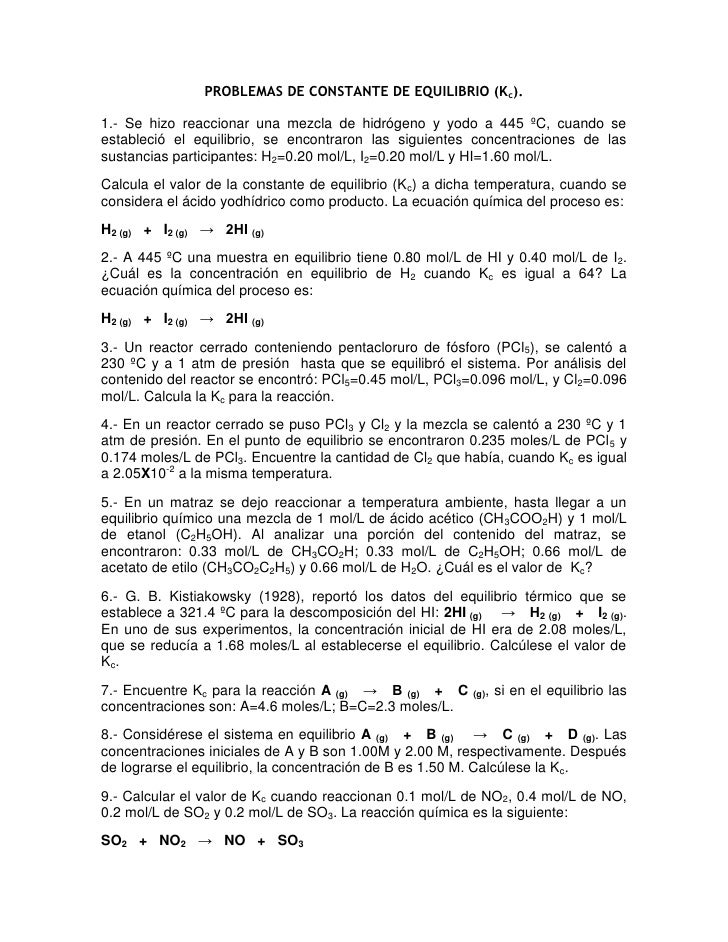Successfully reported this slideshow.
We use your LinkedIn profile and activity data to personalize ads and to show you more relevant ads. You can change your ad preferences anytime.Upcoming SlideShare
Loading in …5
×

# PROBLEMAS DE CONSTANTE DE EQUILIBRIO Kc

41,401 views

Published on

EJERCICIOS DEL TEMA CINÉTICA QUÍMICA DE LA MATERIA DE QUÍMIA II DE PREPARATORIA...COLEGIO AMERICANO DE SAN CARLOS...tango/tango

• Full Name
Comment goes here.

Are you sure you want to Yes No
Your message goes here• Be the first to comment

### PROBLEMAS DE CONSTANTE DE EQUILIBRIO Kc

1. 1. PROBLEMAS DE CONSTANTE DE EQUILIBRIO (Kc). 1.- Se hizo reaccionar una mezcla de hidrógeno y yodo a 445 ºC, cuando se estableció el equilibrio, se encontraron las siguientes concentraciones de las sustancias participantes: H2=0.20 mol/L, I2=0.20 mol/L y HI=1.60 mol/L. Calcula el valor de la constante de equilibrio (Kc) a dicha temperatura, cuando se considera el ácido yodhídrico como producto. La ecuación química del proceso es: H2 (g) + I2 (g) → 2HI (g) 2.- A 445 ºC una muestra en equilibrio tiene 0.80 mol/L de HI y 0.40 mol/L de I2. ¿Cuál es la concentración en equilibrio de H2 cuando Kc es igual a 64? La ecuación química del proceso es: H2 (g) + I2 (g) → 2HI (g) 3.- Un reactor cerrado conteniendo pentacloruro de fósforo (PCl5), se calentó a 230 ºC y a 1 atm de presión hasta que se equilibró el sistema. Por análisis del contenido del reactor se encontró: PCl5=0.45 mol/L, PCl3=0.096 mol/L, y Cl2=0.096 mol/L. Calcula la Kc para la reacción. 4.- En un reactor cerrado se puso PCl3 y Cl2 y la mezcla se calentó a 230 ºC y 1 atm de presión. En el punto de equilibrio se encontraron 0.235 moles/L de PCl 5 y 0.174 moles/L de PCl3. Encuentre la cantidad de Cl2 que había, cuando Kc es igual a 2.05X10-2 a la misma temperatura. 5.- En un matraz se dejo reaccionar a temperatura ambiente, hasta llegar a un equilibrio químico una mezcla de 1 mol/L de ácido acético (CH 3COO2H) y 1 mol/L de etanol (C2H5OH). Al analizar una porción del contenido del matraz, se encontraron: 0.33 mol/L de CH3CO2H; 0.33 mol/L de C2H5OH; 0.66 mol/L de acetato de etilo (CH3CO2C2H5) y 0.66 mol/L de H2O. ¿Cuál es el valor de Kc? 6.- G. B. Kistiakowsky (1928), reportó los datos del equilibrio térmico que se establece a 321.4 ºC para la descomposición del HI: 2HI (g) → H2 (g) + I2 (g). En uno de sus experimentos, la concentración inicial de HI era de 2.08 moles/L, que se reducía a 1.68 moles/L al establecerse el equilibrio. Calcúlese el valor de Kc . 7.- Encuentre Kc para la reacción A (g) → B (g) + C (g), si en el equilibrio las concentraciones son: A=4.6 moles/L; B=C=2.3 moles/L. 8.- Considérese el sistema en equilibrio A (g) + B (g) → C (g) + D (g). Las concentraciones iniciales de A y B son 1.00M y 2.00 M, respectivamente. Después de lograrse el equilibrio, la concentración de B es 1.50 M. Calcúlese la Kc. 9.- Calcular el valor de Kc cuando reaccionan 0.1 mol/L de NO2, 0.4 mol/L de NO, 0.2 mol/L de SO2 y 0.2 mol/L de SO3. La reacción química es la siguiente: SO2 + NO2 → NO + SO3
2. 2. 10.- En un matraz de reacción se había logrado el siguiente estado de equilibrio: 0.3 mol/L de CO, con 0.2 mol/L de Cl2 y 0.8 mol/L de COCl2 (fosgeno). Calcular el valor de Kc para esta reacción: CO + Cl2 → COCl2 11.- Encontrar la Kc si las concentraciones en equilibrio son: 0.3 mol/L de N2, 0.9 mol/L de H2 y 1.4 mol/L de NH3. La reacción es: N2 (g) + 3H2 (g) → 2NH3 (g) 12.- Calcúlese la concentración de A en equilibrio para el sistema gaseoso homogéneo A + B → C + D, si Kc = 0.36 y A y B están inicialmente a una concentración 2.00 M. 13.- Considérese el siguiente sistema en equilibrio a 1000 ºC: H2 (g)+ I2 (g)→ 2HI (g) y Kc=16.0 Si 2.00 moles de H2 y 2.00 moles de I2 se introducen en un recipiente de 500 mL y se calientan a 1000 ºC hasta establecer el equilibrio, ¿Cuántas moles de cada sustancia estarán presentes? 14.- Escríbase la expresión de la constante de equilibrio, Kc, para cada uno de los siguientes sistemas gaseosos en equilibrio: a) 2ClO ↔ Cl2 + O2 b) 2NO + Cl2 ↔ 2NOCl c) CH4 4F2 ↔ CF4 + 4HF d) 2NO2 ↔ 2NO + O2 e) 3O2 ↔ 2O3 f) 2O3 ↔ 2O3 h) 2SO3 ↔ 2SO2 + O2 i) CH3CO2C2H5 (l) + H2O (l) ↔ CH3CO2H (l) + C2H5OH (l) j) NH2CO2NH4 (s) ↔ 2NH3 (g) + CO2 (g) k) CH3CO2H (l) + C2H5OH (l) ↔ CH3CO2C2H5 (l) + H2O (l)